Question 1Question 2Question 3Question 4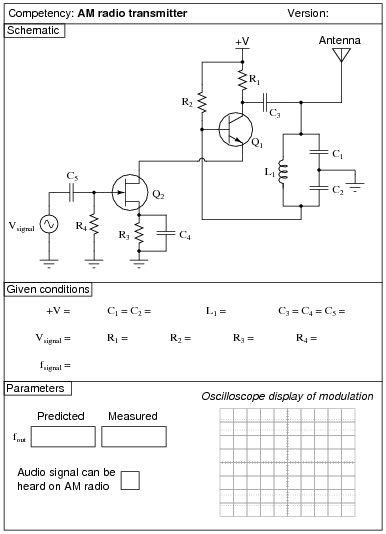Question 5Question 6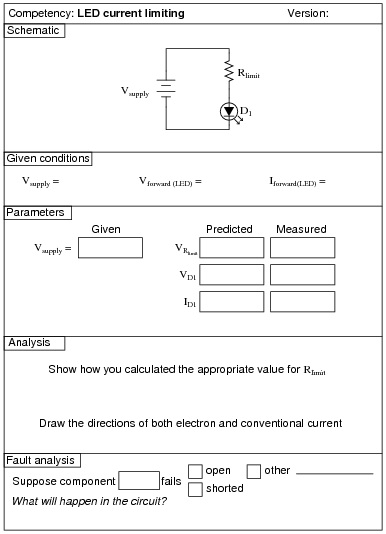Question 7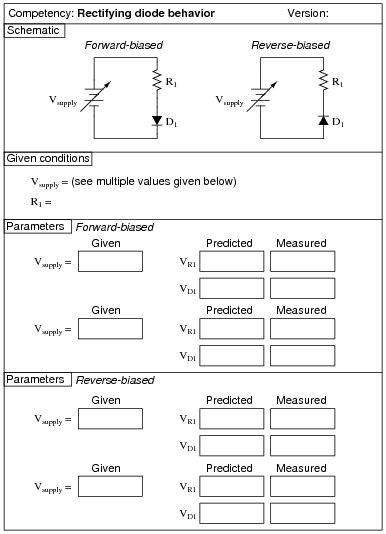Question 8Question 9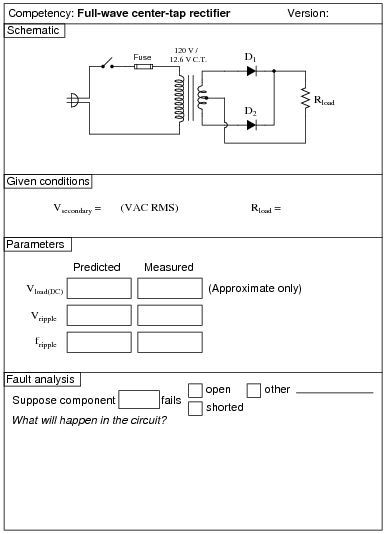Question 10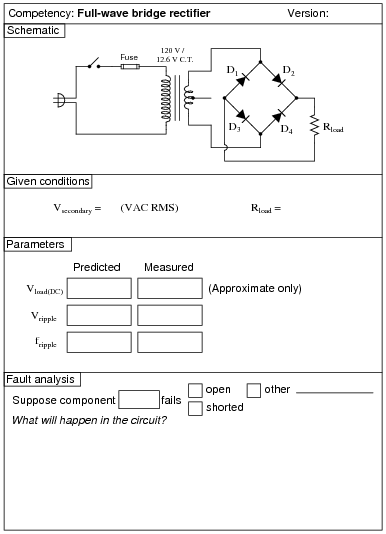Question 11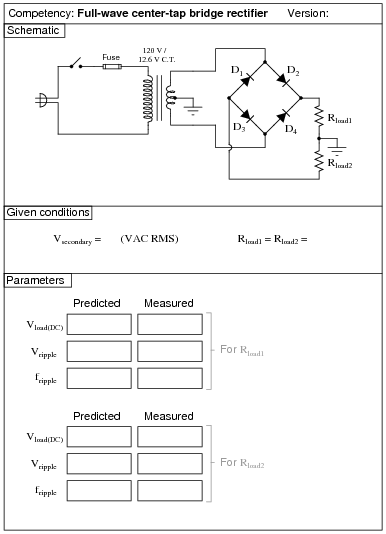Question 12Question 13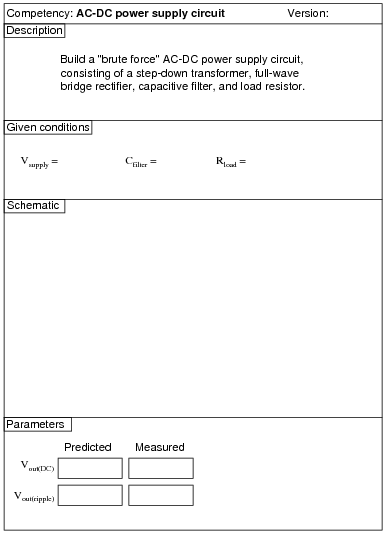Question 14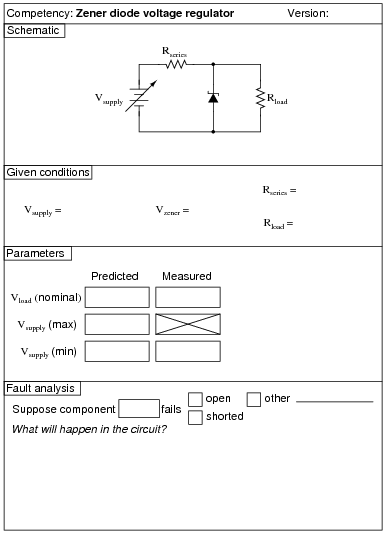The Vsupply (min) parameter is the minimum voltage setting that Vsupply may be adjusted to with the regulator circuit maintaining constant load voltage at Rload. Vsupply (max) is the maximum voltage that Vsupply may be adjusted to without exceeding the zener diode’s power rating. Vload (nominal) is simply the regulated voltage output of the circuit under normal conditions.

Question 15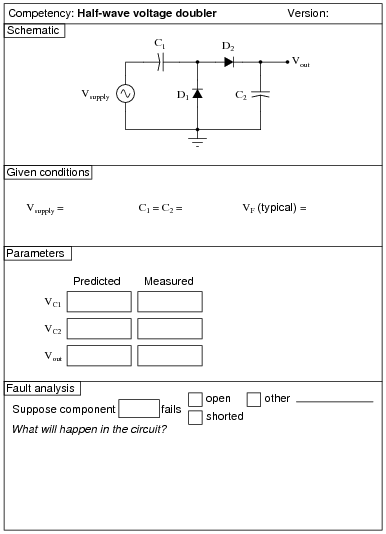Question 16Question 17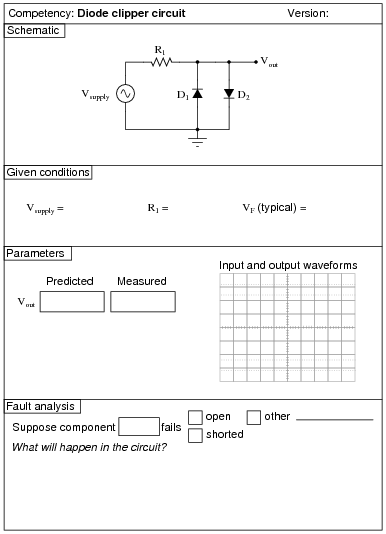Question 18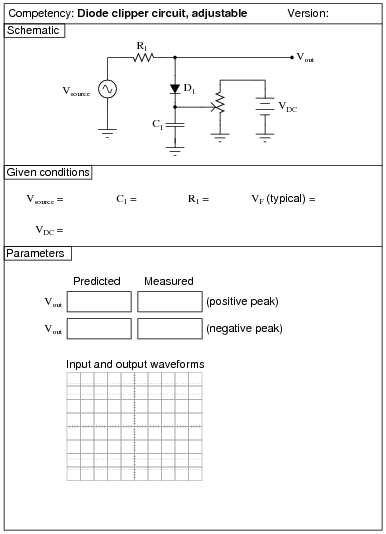Question 19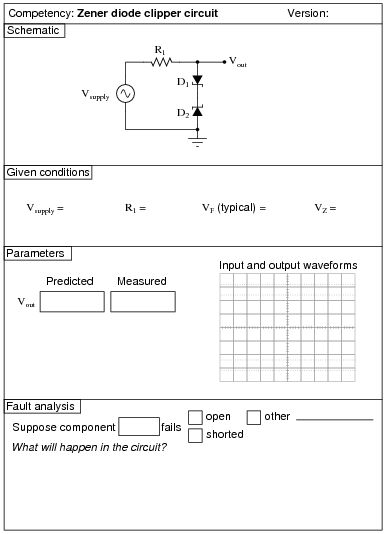Question 20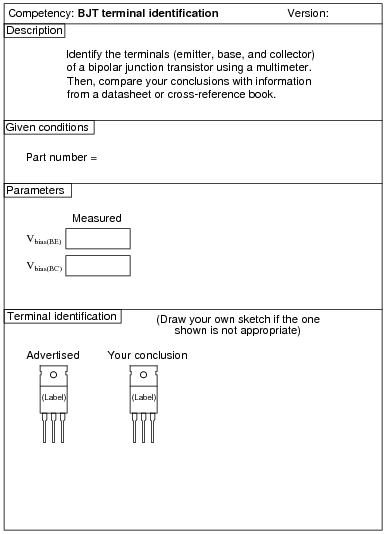Question 21Question 22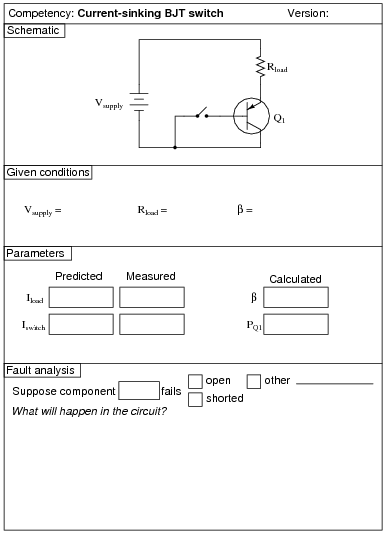Question 23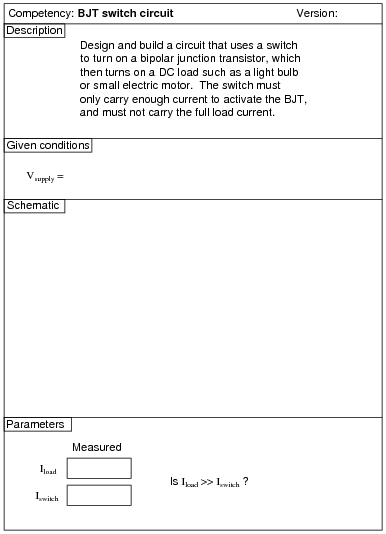Question 24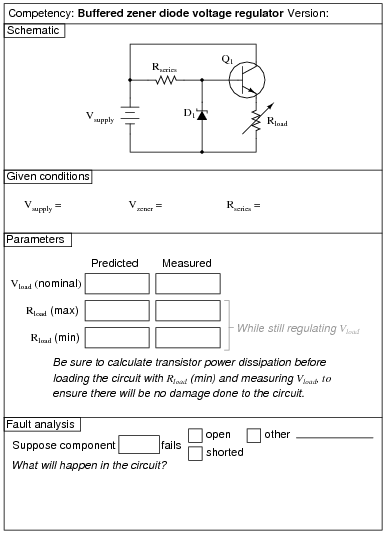The Rload (max) and Rload (min) parameters are the maximum and minimum resistance settings that Rload may be adjusted to with the regulator circuit maintaining constant load voltage. Vload (nominal) is simply the regulated voltage output of the circuit under normal conditions.

Question 25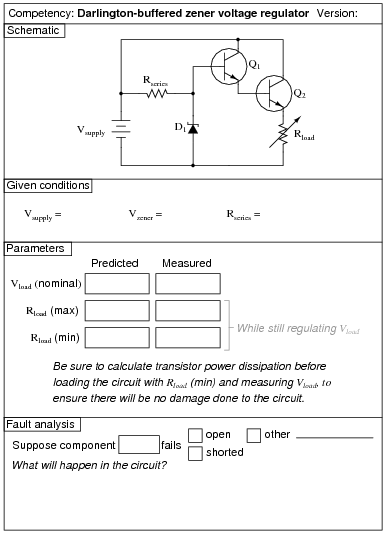The Rload (max) and Rload (min) parameters are the maximum and minimum resistance settings that Rload may be adjusted to with the regulator circuit maintaining constant load voltage. Vload (nominal) is simply the regulated voltage output of the circuit under normal conditions.

Question 26Question 27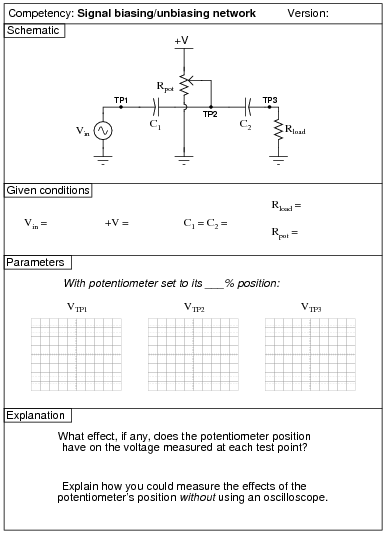Question 28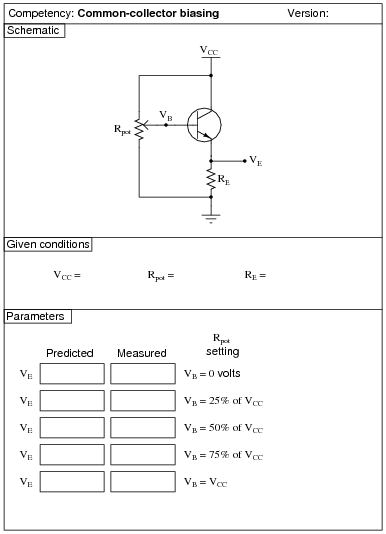Question 29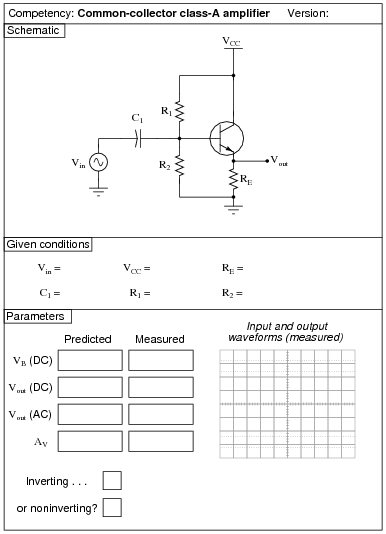Question 30Question 31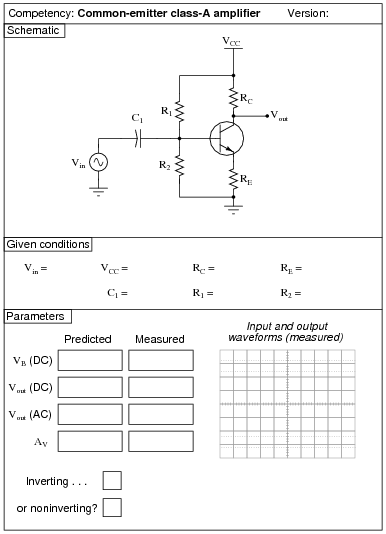Question 32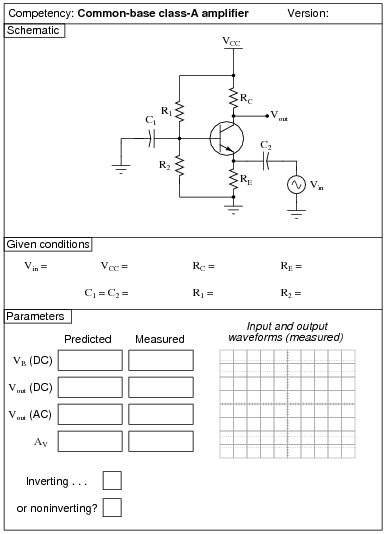Question 33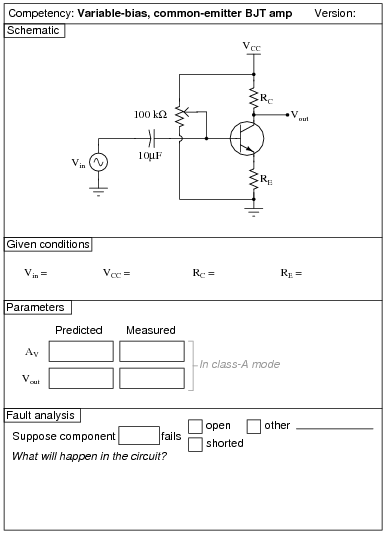Question 34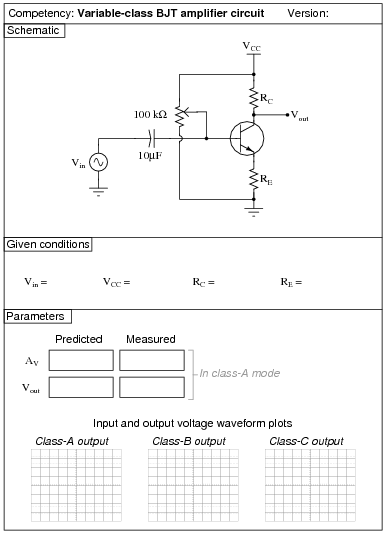Question 35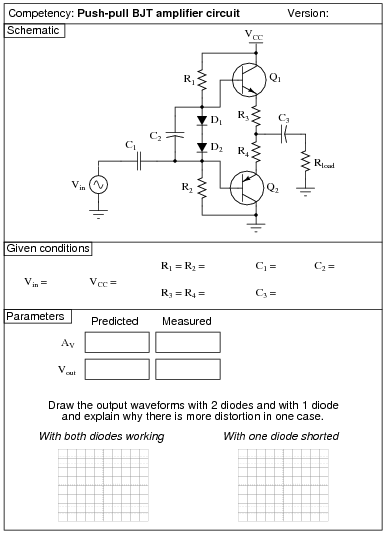Question 36Question 37Question 38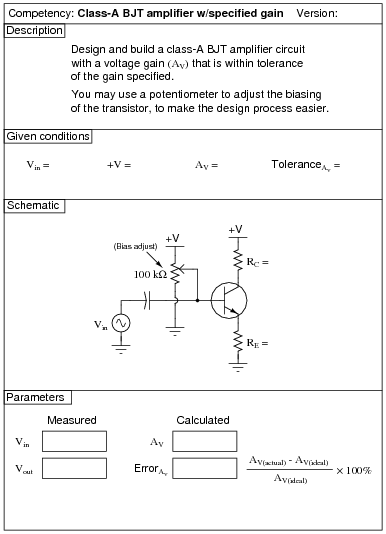Question 39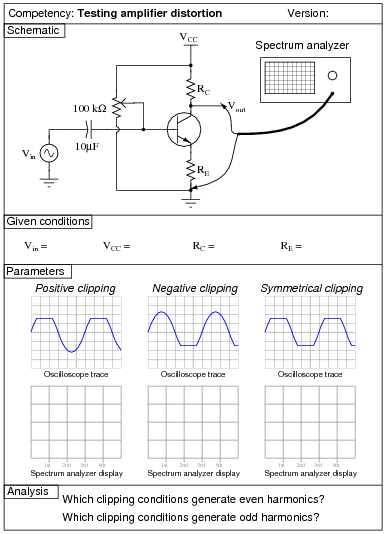Question 40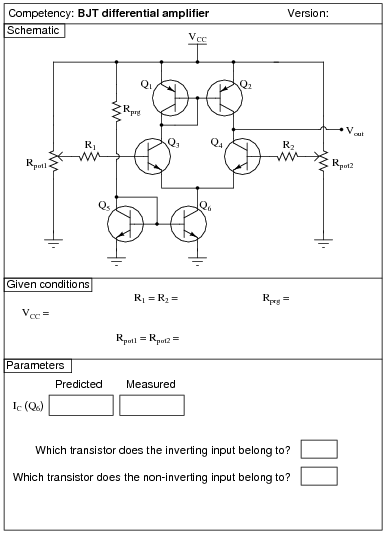Question 41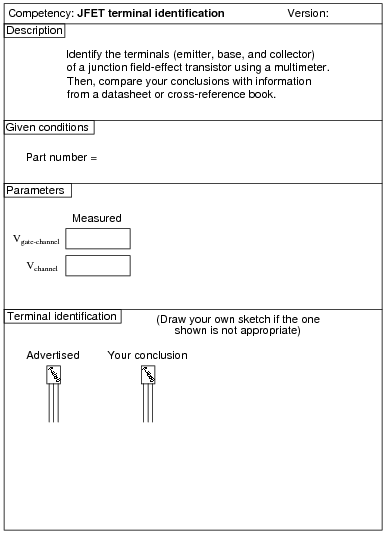Question 42Question 43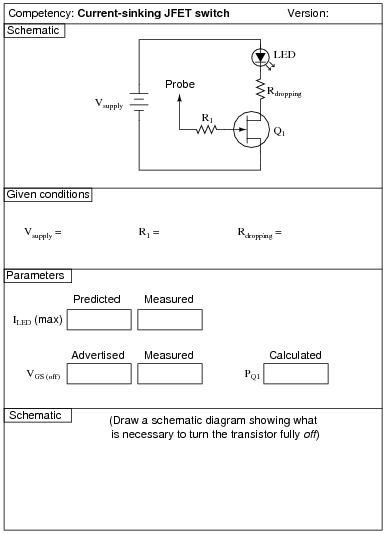Question 44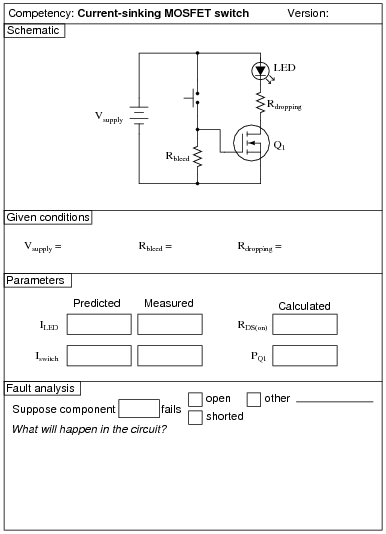Question 45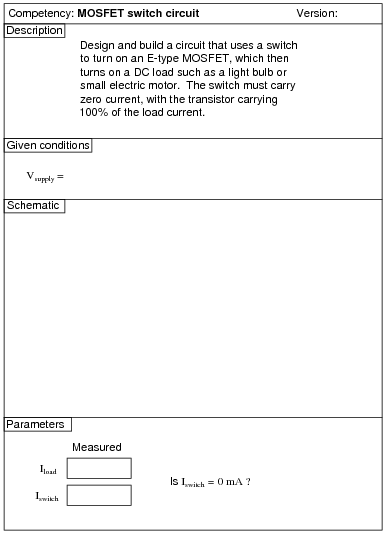Question 46Question 47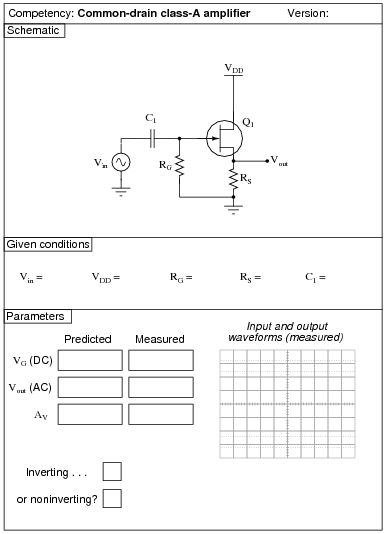Question 48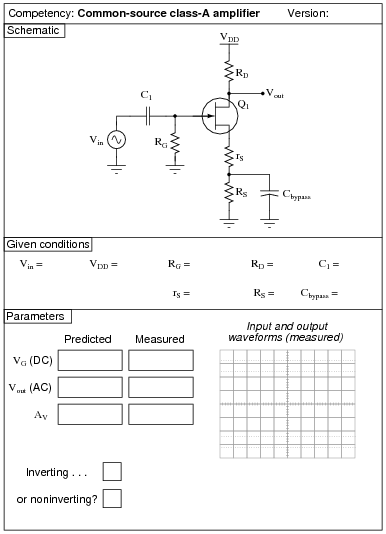Question 49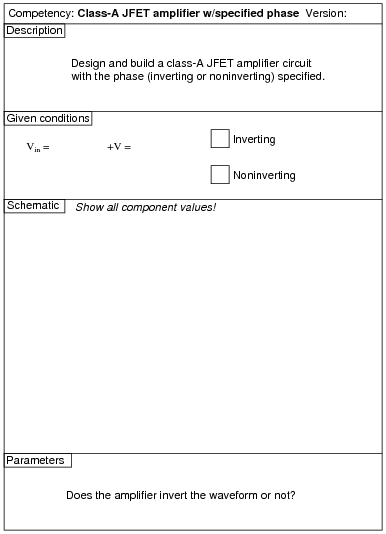Question 50Question 51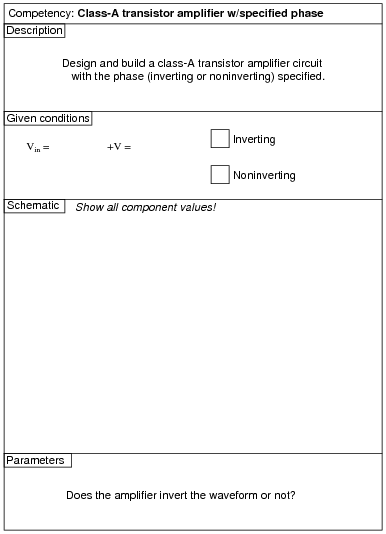Question 52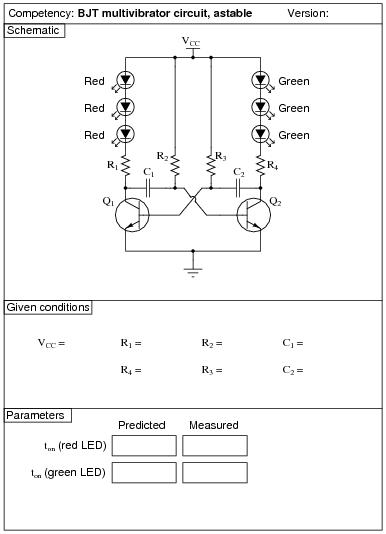Question 53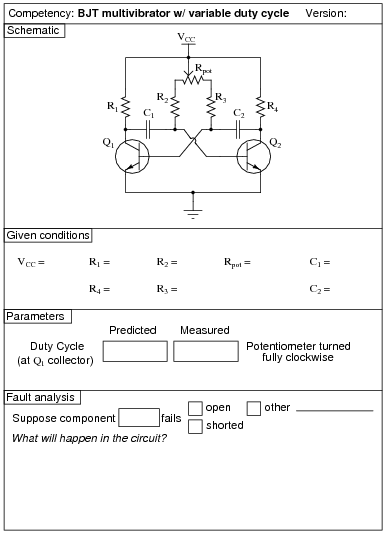Question 54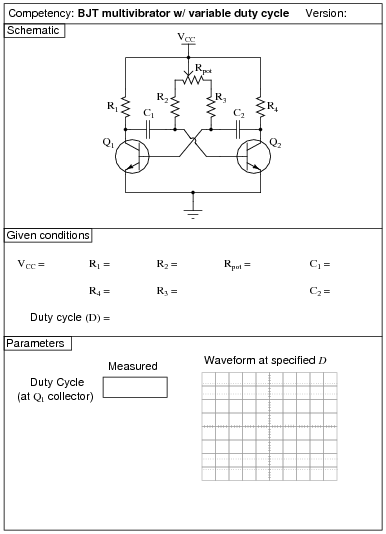Question 55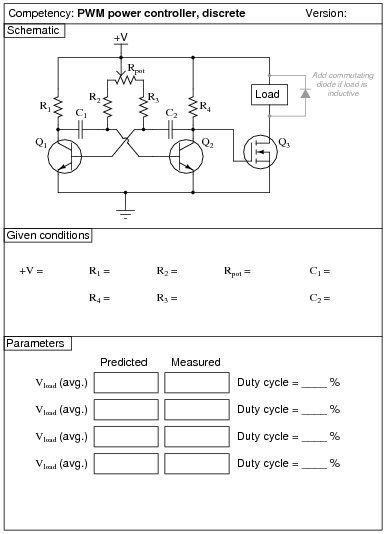Question 56Question 57Question 58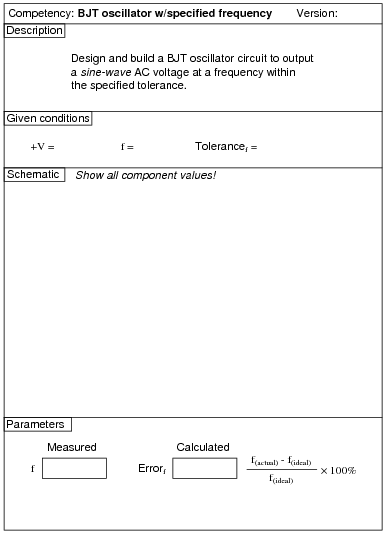Question 59Question 60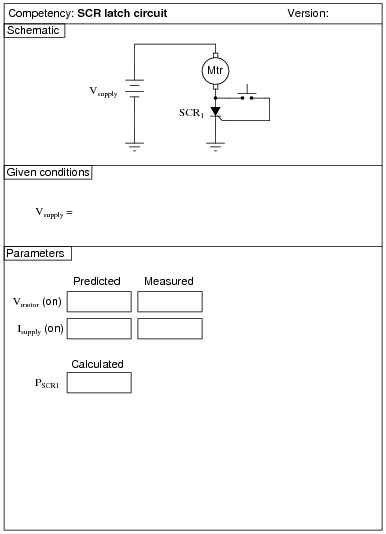Question 61Question 62
 (Template)#### Related Tools:

Published under the terms and conditions of the Creative Commons Attribution License